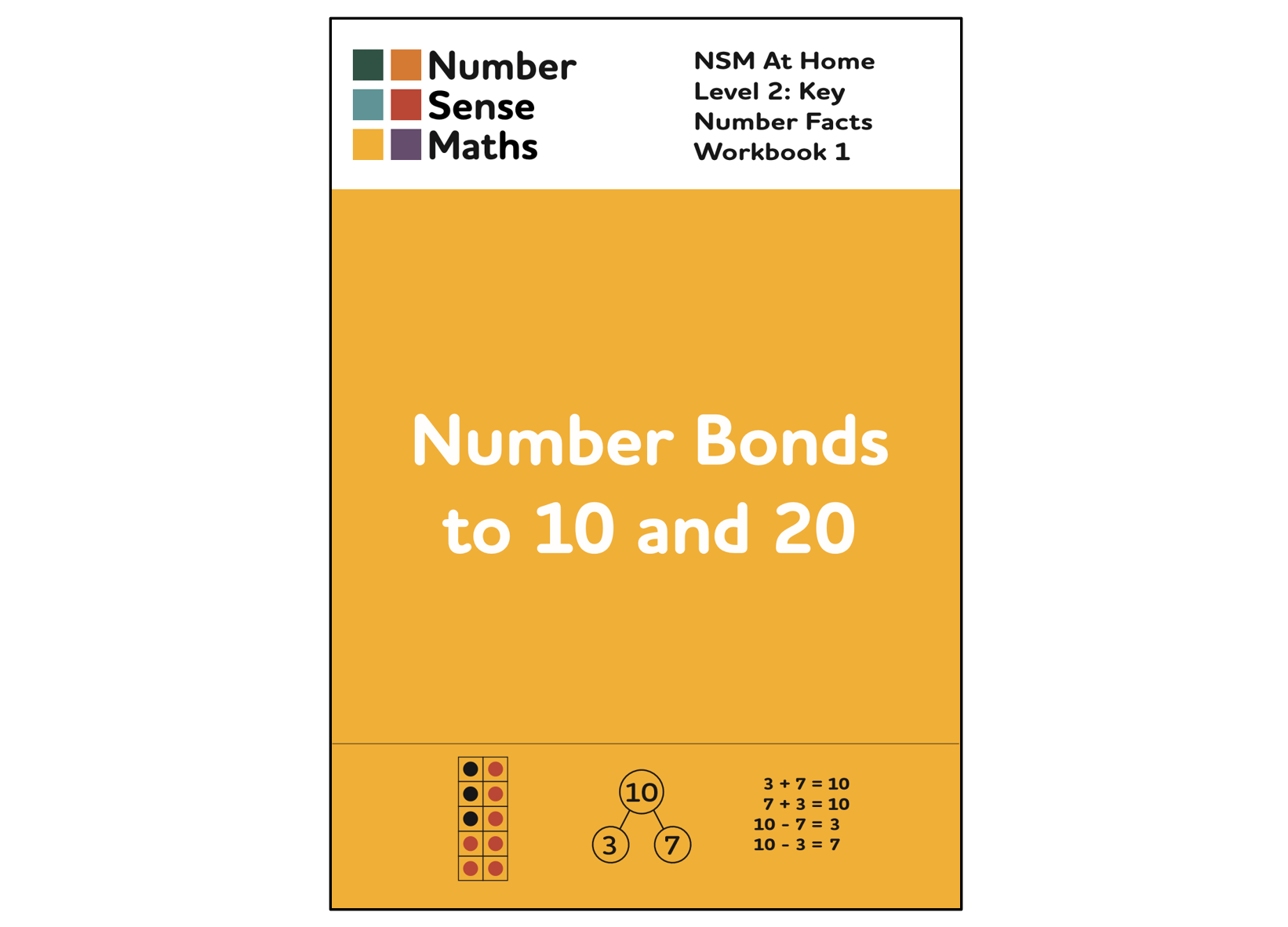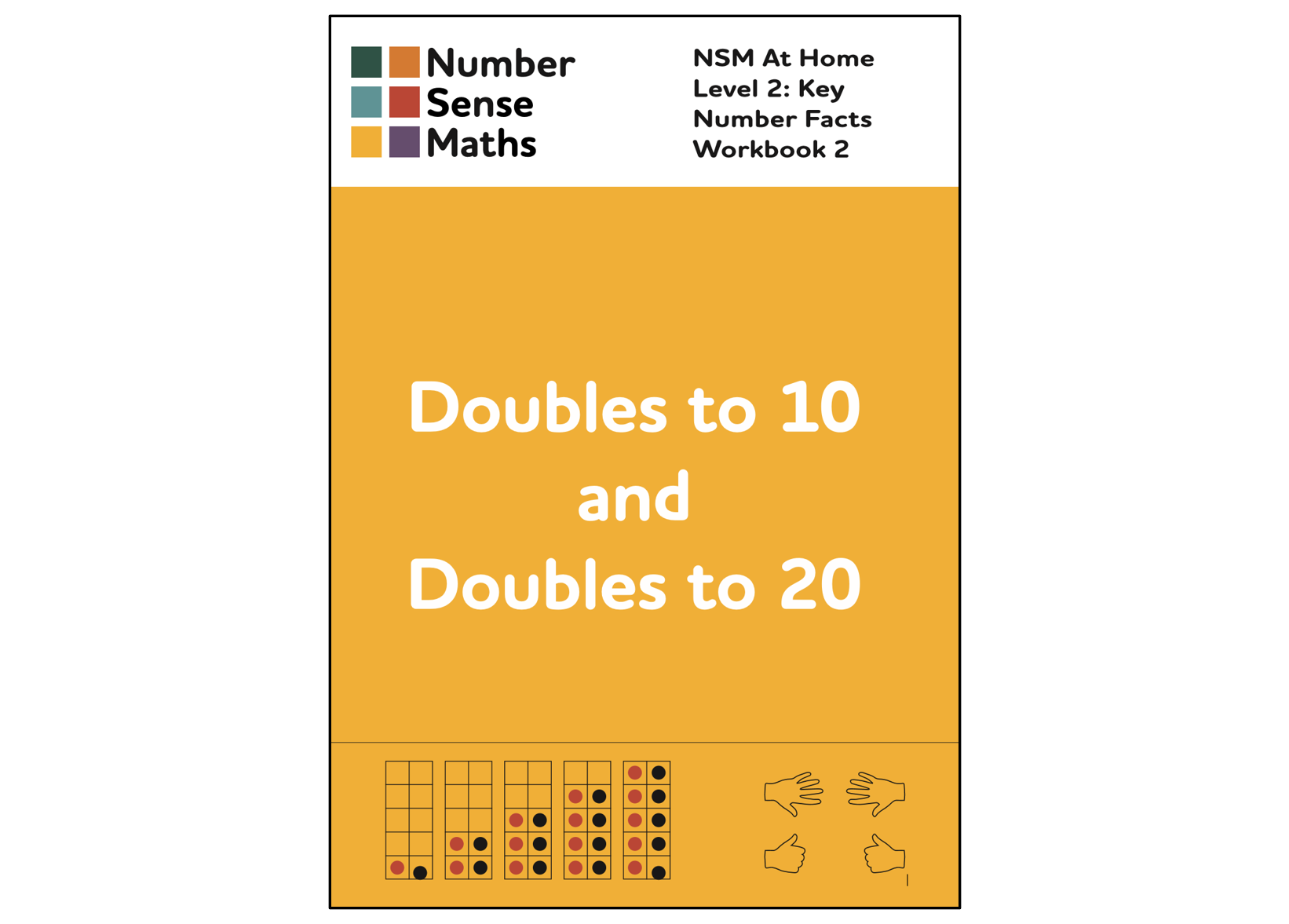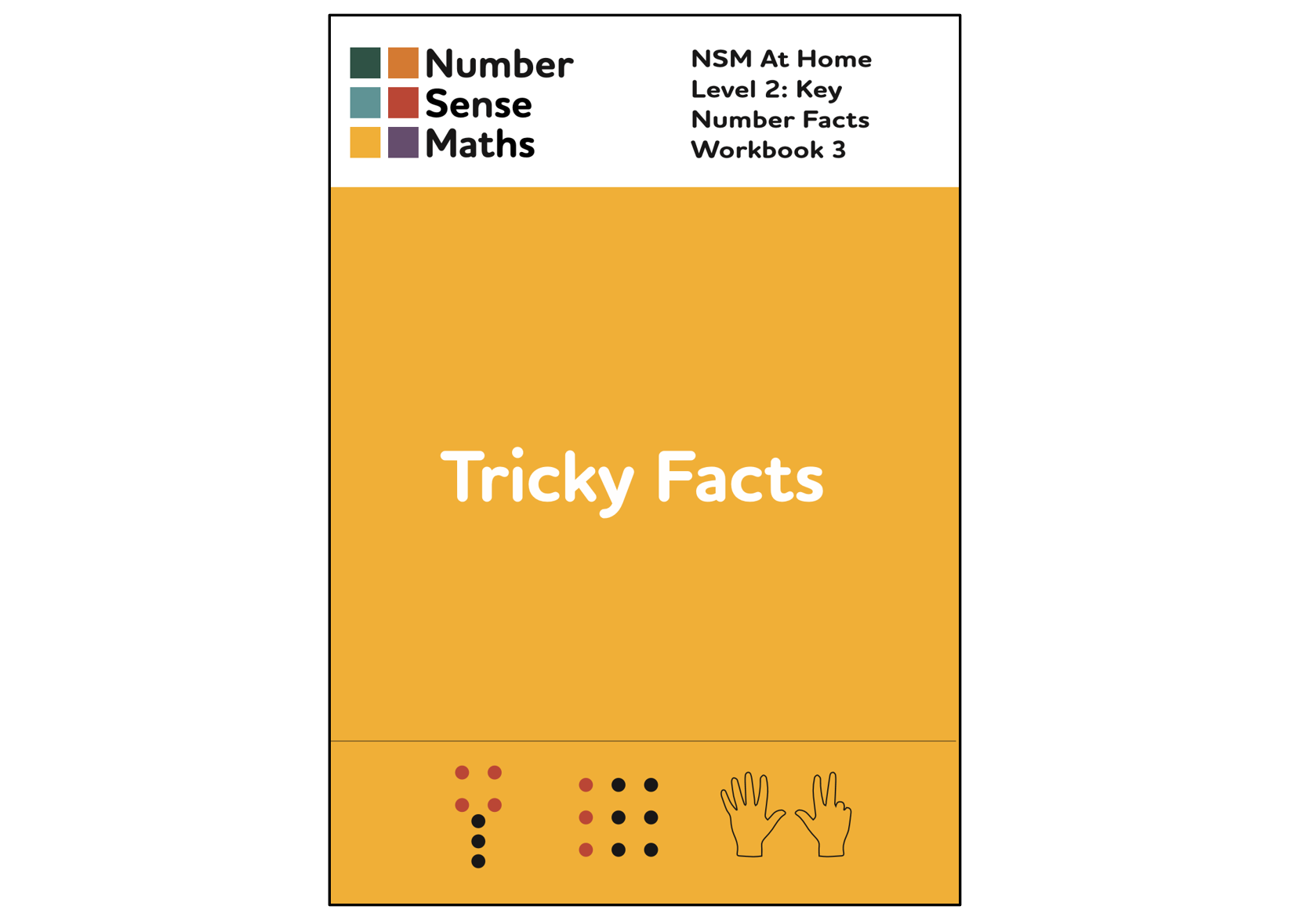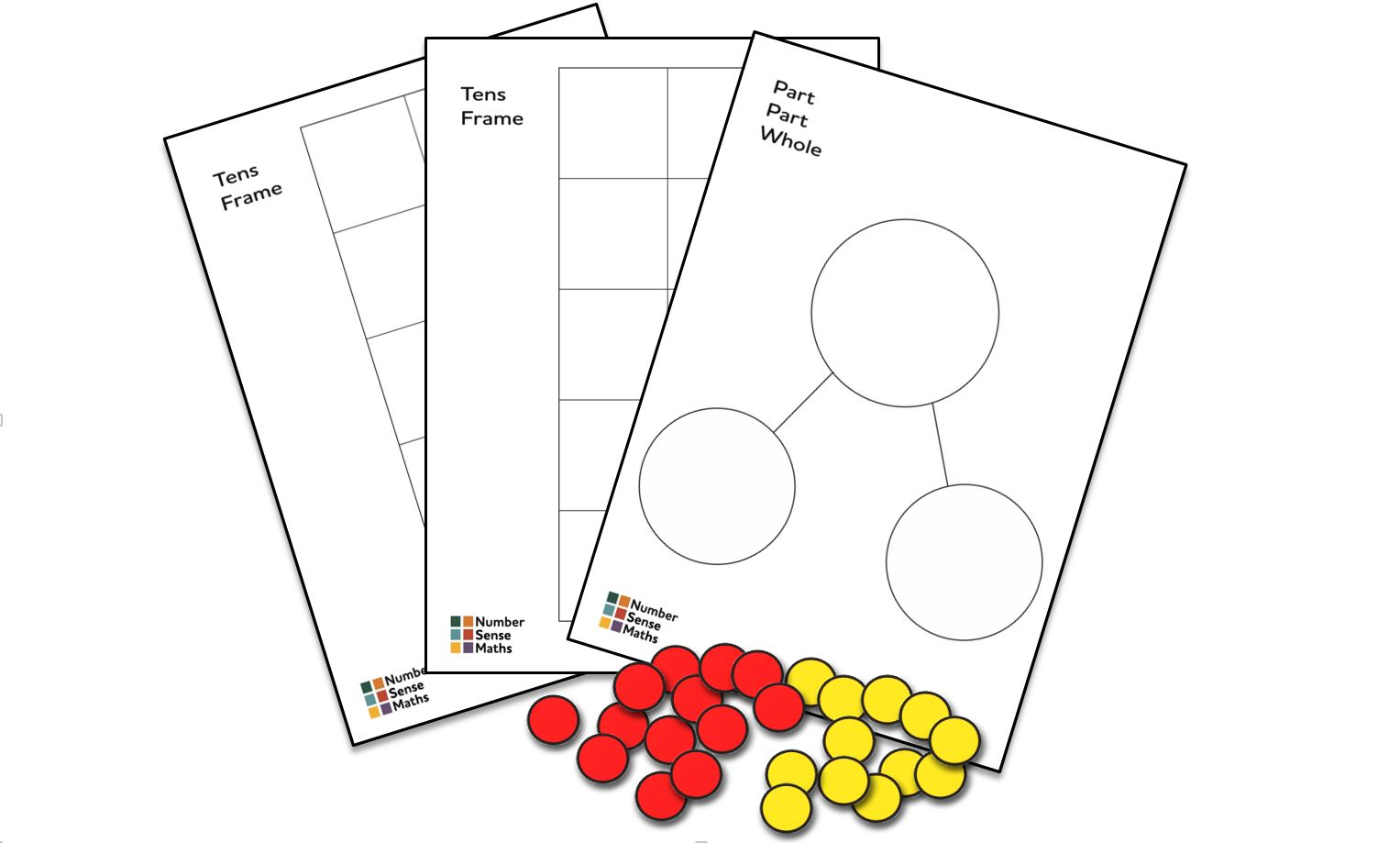# Key Number Facts

The Key Number Facts workbooks teach the key addition and subtraction facts your child needs to know. With these number facts secured your child can move on to calculating: using what they know to work out what they don't yet know.

With three engaging workbooks, supported by flashcards, mats and counters, learning is practical, visual and fun. Guidance for tutors and parents on how to support and maximise learning is provided throughout, including on how to use the animations in the Home Learning Zone to master key concepts and to gently check progress.

## Workbooks

1. Number Bonds

Age 5+35 pages

Number bonds to 10 are pairs of numbers that add to 10, like 7 and 3. Number bonds to 20 are pairs of numbers that add to 20, like 17 and 3. We can use each number bond to 10 to solve four related addition and subtraction facts without counting. For example, with 7 and 3 we can solve 7 + 3, 3 + 7, 10 - 7 and 10 - 3.

If we understand 'Ten and A Bit' numbers well (taught in Level 1: Number and Quantity), we can extend our knowledge of number bonds to 10 to number bonds to 20 without learning any new pairs. 17 is made up of one set of 10 and 7 ones. When know that 7 bonds with 3 to make 10, it must be true that 17 bonds with 3 to make 20. We are just adding 3 to the 7 to make the second set of 10.

This workbook teaches number bonds to 10 and 20 visually and practically through a range of activities, exercises, animations and flashcards.

2. Doubles

Age 5+31 pages

Doubles to 10 are doubles of the numbers 1 to 5. Doubles to 20 are doubles of the numbers 6 to 10. We can use double facts, and their corresponding halves, to solve related addition and subtraction equations without counting. For example, we can use double 7 to solve 14 - 7, and 7 + 7.

Children need to be so familiar with their doubles that when they see an equation such as 8 - 4, they immediately think doubles and recall the relevant double fact, rather than counting to solve the equation.

This workbook teaches doubles using two key visual models: tens frames are used for doubles to 10, and 'Five and A Bit' hands are used for doubles to 20. When these double facts are known they can also be used to calculate near double equations, for example, 8 + 9 can be solved as double 8 plus 1. This calculation approach is taught in Level 3 once the doubles facts are secure.

3. Tricky Facts

Age 5+15 pages

Tricky facts are some of the core addition and subtraction facts that are difficult to solve. Since they are difficult to solve, it helps children to be able to recall these facts rather than getting bogged down trying to solve them from scratch, often by counting in ones or on fingers.

This workbook teaches teaches 12 tricky facts. They are taught using 3 key visual images: the 7 tree, the 9 square and 8 shown on 'Five and A Bit' hands. The visual images are then each related to the four addition and subtraction facts that they enable children to solve. For example, the 7 tree image shows us that 7 is made up of 4 and 3. This helps us solve 4 + 3, 3 + 4, 7 - 4, and 7 - 3.

As with other known facts, when knowledge of these tricky facts is secure we can use them not only to solve tricky fact equations, but also to calculate near facts that we don't yet know.

## Also Included

FlashcardsA set of 76 flashcards covering number bonds to 10 and 20, doubles to 10 and 20, and the key tricky fact equations. Use at the end of each chapter and little and often at the end of the workbook, both to practise the key facts and to gently check what your child has and hasn't yet mastered.

Resource PackPractical and visual resources to help your child learn their key number facts. Includes a blank A4 part part whole model, two blank A4 tens frame models, and 25 double sided counters. Use these to complete the activities and exercises in the workbooks.